Reach Us+44-1522-440391
Intrinsically Ties Adjusted Sign Test by Ranks | OMICS International
Journal of Biometrics & Biostatistics

Like us on:

All submissions of the EM system will be redirected to Online Manuscript Submission System. Authors are requested to submit articles directly to Online Manuscript Submission System of respective journal.

# Intrinsically Ties Adjusted Sign Test by Ranks

Oyeka ICA*

Department of Applied Statistics, Nnamdi Azikiwe University, Awka, Nigeria

*Corresponding Author:
Oyeka ICA
Professor of Statistics
Department of Applied Statistics
Nnamdi Azikiwe University
Awka, Nigeria
Tel: +238052563956
E-mail: [email protected]

Received date: June 25, 2012; Accepted date: November 05, 2012; Published date: November 10, 2012

Citation: Oyeka ICA (2012) Intrinsically Ties Adjusted Sign Test by Ranks. J Biom Biostat 3:154. doi:10.4172/2155-6180.1000154

Copyright: © 2012 Oyeka ICA. This is an open-access article distributed under the terms of the Creative Commons Attribution License, which permits unrestricted use, distribution, and reproduction in any medium, provided the original author and source are credited.

Visit for more related articles at Journal of Biometrics & Biostatistics

#### Abstract

This paper proposes and discusses a non-parametric statistical method for the analysis of paired or matched sample data based on ranks rather than on the raw scores themselves. The proposed method intrinsically and structurally adjusts the test statistic for the possible presence of tied observations between the sampled populations and hence obviates the need to require these populations to be continuous. The number k used in the ranking may be any real number and does not affect the result of the analysis. The proposed method can be used with both numeric and nonnumeric measurements on as low as the ordinal scale and is easily modified for use with one sample data. The method is illustrated with some data and shown to compare favorably with the usual sign test and the Wilcoxon signed rank sum test in cases where these two methods may be equally used in data analysis.

#### Keywords

Wilcoxon; Sign test; Non-parametric; Ranks; Structurally

#### Introduction

If a researcher has paired sample data that satisfy the usual assumptions of normality and continuity, then the parametric pairsample t-test may be used to determine whether the sampled populations have equal means or some other measure of central tendency. However, if these assumptions are not satisfied by the data, then the parametric t-test cannot be properly used for data analysis. Use of non-parametric methods is then indicated and preferable. Non-parametric methods that readily suggest themselves include the ordinary sign test for paired samples and the Wilcoxon Signed Rank Sum test [1-3]. The problem with these sign tests is that they require the data being analyzed to be continuous numeric measurements, a requirement that often reduces the power of the tests if they are tied observations between the sampled populations. Methods for adjusting for ties if they occur across samples are available but often difficult to use in practice. Some of these methods include ignoring the tied observations and reducing the total sample size accordingly; randomly assigning the tied observations to either one or the other portion of the data dichotomized in some way by some chosen measure of interest; or assigning them their mean ranks [1,3-5]. These approaches do not however always recommend themselves because of the reduction in the power of the resulting test statistic especially if the tied observations are not few. Siegel  has developed a method of estimating the effect of ties and correcting them in the test statistic but the calculations are often cumbersome and tedious to use in practical applications. Oyeka and others  have developed a method that intrinsically and structurally adjusts the test statistic for the possible presence of ties in the data and hence obviates the need to require the sampled populations to be continuous. However, this method cannot be used without modifications to analyze non-numeric measurements on the ordinal scale. Although the method, because it adjusts for ties, has been shown to be more powerful than the ordinary sign test and the Wilcoxon Signed Rank Sum test, it nevertheless has its limitations in that it is based on the raw data rather than on the ranks of the paired observations themselves, an approach that is expected to further increase the power of the test . In this paper, we propose to develop a modified sign test based on the ranks of the paired sample observations rather than only on the observations themselves and which intrinsically and structurally adjusts for any possible ties in the data, and is available for use with measurements on as low as the ordinal scale whether numeric or non-numeric.

The proposed method

Let (xi1, xi2) be the ith pair of observations randomly drawn from populations X1 and X2 for i=1, 2,…, n. Populations X1 and X2 should be measurements on at least the ordinal scale but they may or may not be:

(1) Continuous; (2) Normally distributed; (3) Numerical data or (4) Independent.

Interest here is to develop a statistical method for the analysis of paired sample data that may be non-numeric measurements on at least the ordinal scale using ranks assigned to these measurements. The proposed method makes provisions for the possible presence of any tied observations between the sampled populations.

Now let xi1 be assigned the rank ri1= k, k-0.5, or k-1 if xi1 is a higher (larger), the same (equal), or lower (smaller) score or observation than xi2. Similarly let xi2 be assigned the ri2= k, k-0.5, or k-1 if xi2 is a higher (larger), the same (equal), or lower (smaller) score or observation than xi1 for i=1, 2,…, n where (xi1, xi2 ) is the ith pair of sample observations and k is any real number.

Now let: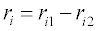(1)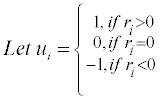(2)

for i=1, 2,..,n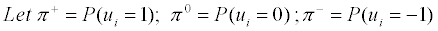(3)

Where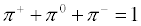(4)

Define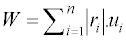(5)

That is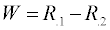(6)

Where R.1 and R.2 are respectively the sums of the ranks assigned to sample observations from populations X1 and X2.

Now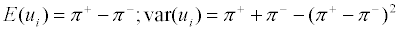(7)

Also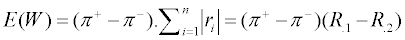(8)

and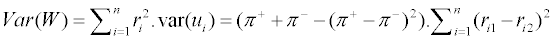That is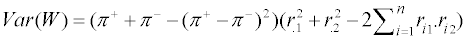(9)

Where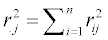, is the sum of squares of the ranks assigned to sample observations from population Xj; j = 1, 2.

Now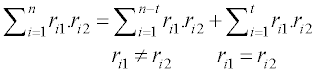Where t is the number of tied observations between populations X1 and X2

That is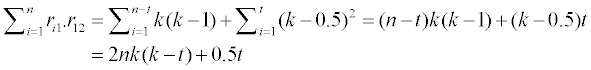Substituting in equation 9 we have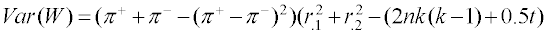(10)

Alternatively,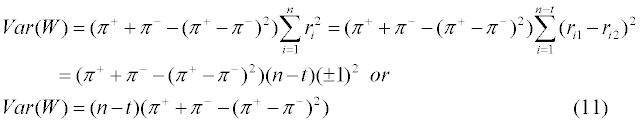which is independent of the real number k:

In fact it is noted that: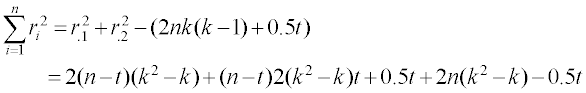That is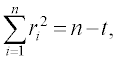which provides an additional proof for the simplified version of the variance of W shown in equation 11.

Note that π+0 and π and are respectively the probabilities that in a randomly selected pair of observations, the observation drawn from population X1 is on the average higher or greater than the same as (equal to) or lower (smaller) than the observation drawn from population X2.

The sample estimates of these probabilities are respectively: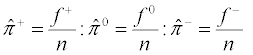(12)

where f+, f0, and f- are respectively the number of 1's,0's and -1's in the frequency distribution of the n values of these numbers in ui, i=1,2,...,n. A null hypothesis that is often of general interest is that the difference between the medians of the sampled populations is some constant value. In other words, a null hypothesis that may be tested is that the difference between the probability that the observations from one of the sampled populations is on the average greater than the observation from the other sampled population and the probability that it is less is some constant value β0, say.

Notationally, the null hypothesis may be expressed as: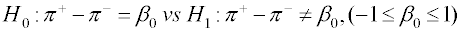(13)

This null hypothesis may be tested using the test statistic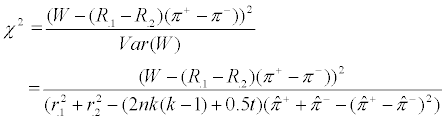(14)

or simply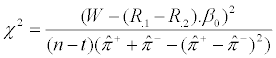(15)

Which under H0 has approximately the chi-square distribution with 1 degree of freedom for sufficiently large n. H0 is rejected at α the level of significance is 0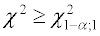(16)

otherwise H0 is accepted.

In particular, under the null hypothesis usually tested in paired sample problems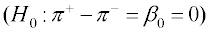, equation (14) simplifies to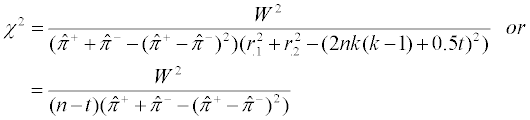(17)

The modified sign test W has been shown to be more efficient and hence more powerful than the usual Wilcoxon Signed Rank Sum Test T+  .However, the proposed modified sign test by ranks which may for the moment be denoted by Wr is also more efficient than the modified sign test statistic W, based on only raw scores. To show this, we note that the relative efficiency of Wr to W is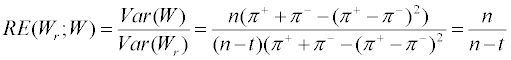So that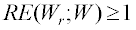(18)

for all t ≥ 0

Hence, for all sample sizes, the modified sign test by ranks is more efficient and thus more powerful than the corresponding modified sign test based on only raw scores whenever there are tied observations between the sampled populations.

Illustrative example

We here illustrate the proposed method with two examples. The first is on ordinal non-numeric scores and the second is on numeric scores as follows.

1. A health insurance company every year assesses the vital signs of its clients for the purpose of determining the annual insurance premium payable. In this process, the company scores its clients from A+ (excellent health) through C (fair health) down to F (poorest health; fail). Persons with excellent health pay the lowest annual health premium while clients with very poor score pay the highest annual premium. The scores earned by a random sample of 15 clients of this health insurance company during the past two consecutive years are as follows:

 Client No 1 2 3 4 5 6 7 8 9 10 11 12 13 14 15 Year 1 score A– A+ D B– A– B F A– A– C+ A+ E F B+ A+ Year 2 score F A– F E B– C+ F B+ C A B– D E B+ C+

2. A random sample of members of each of 15 newly married couples (husband and wife) are asked to state their preferred family sizes (desired number of children) with the following results:

 Couple No. 1 2 3 4 5 6 7 8 9 10 11 12 13 14 15 Husband 4 1 6 1 7 1 4 2 8 5 4 4 5 5 4 Wife 5 5 5 6 5 9 4 6 8 5 4 5 6 6 4

As noted above, the data of example 1 being ordinal non-numeric measurements may only be analyzed using modified sign tests, using either raw scores or ranks. Thus to analyze the data of example 1 using the proposed method, we assign the rank ri1=k, k-0.5 or k-1 to the letter grade assigned to a client in year 1 if it is higher than, the same as or lower than his grade of him in year 2. Similarly, we assign the rank ri2= k, k-0.5 or k-1 if the grade earned by the client in year 2 is higher, the same as or lower than the grade he earned in year 1.The results are presented in table 1 together with the difference between these ranks and other statistics.

Client No Score in
year 1(xi1)
Score in year 2 (xi2) Ui (1 if yr 1 score is higher than yr 2;0 if yr 1 same as yr 2;-1 if yr 1 is lower than yr2) Rank of
xi1 (ri1)
Rank of
xi2 (ri2)
Diff.
(ri=ri1-ri2)
|ri| .ui ri2
1 A F 1 K K–1 1 1 1
2 A+ A 1 K K–1 1 1 1
3 D F 1 K K–1 1 1 1
4 B E 1 K K–1 1 1 1
5 A B 1 K K–1 1 1 1
6 B C+ 1 K K–1 1 1 1
7 F F 0 K-0.5 K–0.5 0 0 0
8 A B+ 1 K K–1 1 1 1
9 A C 1 K K–1 1 1 1
10 C+ A -1 K–1 K -1 -1 1
11 A+ B 1 K K–1 1 1 1
12 E D -1 K–1 K -1 -1 1
13 F E -1 K–1 K -1 -1 1
14 B+ B+ 0 K–0.5 K–0.5 0 0 0
15 A+ C+ 1 K K–1 1 1 1
Total           7 7 13

Table 1: Ranks assigned to the letter grades of example1 together with values of ui (equation 2).

Interest is to use the proposed method to determine whether the median scores by clients are the same for the two years, that is if clients are likely to pay equal insurance premium for each of the two years (Equation 13, with β0=0). To do this, we have from column 4 of table 1 that f+=10, f0=2 and f=3 so that from Equation 12 we have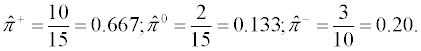Also from Equations 5 and 6 and column 8 of table 1, we have W=10-3=7. From Equation 11 and column 9 of table 1, we have the estimated variance of W as: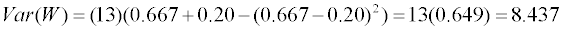The test statistic for our null hypothesis of equal population medians is given from Equation 17 as: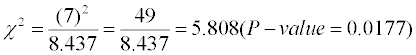which with 1 degree of freedom is highly significant indicating that clients of the health insurance company have different median scores for the two years. If we had used the modified sign test instead based on only raw scores , we would have the relation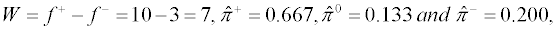so that the corresponding test statistic is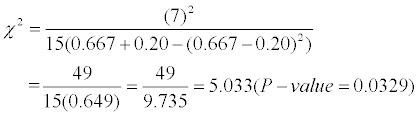which is also statistically significant. However, the relative sizes of the chi-square values and the corresponding attained significance levels show that the proposed modified sign test by ranks is likely to be more powerful than its counterpart based on only raw scores in that the later is likely to accept a false null hypothesis (Type II Error) more frequently than the former test statistic which is able to use more information on the data being analyzed.

Table 2 presents the application of the proposed method to the numeric data of example 2.

Couples Husband Wife Di     Ui
(eqn 2)
Rank of
xi1
Rank of
xi2
Diff (ri=ri1-ri2)
(Eqn. 1)
|ri| .ui ri2 Rank of non-zero absolute diff
xi1 xi2 (xi1-xi2)   (ri1) (ri2) ri=ri1– ri2     r(|di|)
1 4 5 -1 -1 K–1 K -1 -1 1 3
2 1 5 -4 -1 K–1 K -1 -1 1 7.5
3 6 5 1 1 K K–1 1 -1 1 3
4 1 6 -5 -1 K–1 K -1 -1 1 9
5 7 5 2 1 K K–1 1 1 1 6
6 1 9 -8 -1 K–1 K -1 -1 1 10
7 4 4 0 0 K-0.5 K-0.5 0 0 0
8 2 6 -4 -1 K–1 K -1 -1 1 7.5
9 8 8 0 0 K-0.5 K-0.5 0 0 0
10 5 5 0 0 K-0.5 K-0.5 0 0 0
11 4 4 0 0 K-0.5 K-0.5 0 0 0
12 4 5 -1 -1 K–1 K -1 -1 1 3
13 5 6 -1 -1 K–1 K -1 -1 1 3
14 5 6 -1 -1 K–1 K -1 -1 1 3
15 4 4 0 0 K-0.5 K-0.5 0 0 0
Total               -6 10

Table 2: Analysis of family size preferences by couples using modified sign tests.

To apply the proposed method to the numeric data on family size preferences by couples we have from column 5 of table 2 that f+=2, f0=5 and f=8 so that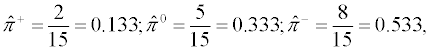also from column 9 and 10 of table 2, we have that W=2-8= –6 and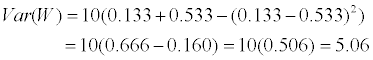Hence, the corresponding test statistic (Equation 17) is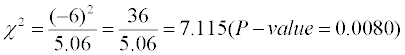which with 1 degree of freedom is highly statistically significant. If we had used the modified sign test based on only raw scores to analyze the data we would have with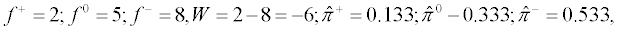that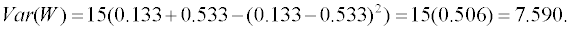.

Hence, the corresponding chi-square test statistic is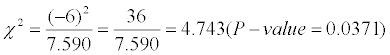which is again statistically significant although not as strongly significant as the one based on ranks. It may be instructive to analyze the numeric data of example 2 using the paired sample parametric t test and the ordinary sign test for comparative purposes. Using the parametric t test, we have that the sample mean difference in couple family size preference is: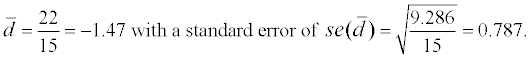The test statistic for the null hypothesis of equal population medians (H0:d0=0) is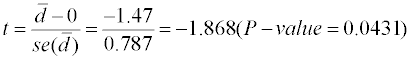with 14 degrees of freedom is statistically significant, although here not as powerful as the modified sign tests. To use the ordinary sign test with the data we note that since they are altogether 5 tied observations between the paired sample observations, the effective sample size is n′ = 2 + 8 =10. Hence, if X is the number of + signs which is here, the number of cases in which husbands have higher family size preferences than wives and if under H0: p=0.50, then with n′ =10 we have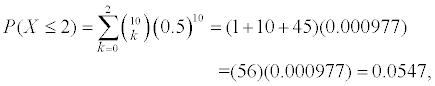which is not now statistically significant, a conclusion that is occasioned by the fact that tied observations are excluded in the analysis. Finally, it may also be instructive to compare the results obtained here using the modified sign test with what would have been obtained if the usual Wilcoxon Sign Rank Sum test is used to analyze the data of example 2. To do this, we rank the non-zero absolute differences |di| of table 2 from the smallest to the largest. The results are shown in column 11 of table 2. From this column, we have that T+ = 3+6 = 9 ,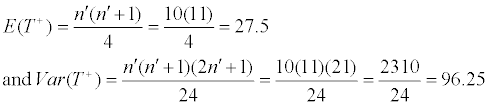Therefore, the corresponding chi-square test statistic for equal population medians is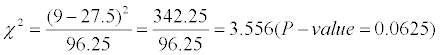which with 1 degree of freedom is not now statistically significant, leading to an acceptance of the null hypothesis of equal family size desires by newly married husbands and wives. Thus, the modified sign test by ranks is more likely to reject a false null hypothesis and accept a true one than the usual Wilcoxon Signed Rank Sum Test by ranks. The problem with the Wilcoxon Signed Rank Sum test unlike the modified sign tests is that it ignores tied observations and is based on only non-zero absolute differences which consequently lead to loss of power.

#### Summary and Conclusion

In this paper, we developed a non-parametric modified sign test for the analysis of paired or matched sample data which accommodates paired ordinal data. The proposed test statistic is intrinsically and structurally adjusted and modified to allow for the possible presence of tied observations between the sampled populations and hence obviate the need to require these populations to be continuous. The number k employed in the ranking of the paired observations may be any real number and does not affect the results of the analysis. The proposed method may be used with both numeric and non-numeric measurements on at least the ordinal scale. The method is also easily modified for use in the analysis of one-sample data. The proposed method is illustrated with some data and shown to be more powerful than the usual sign tests and at least as powerful as the modified sign test based on only raw scores or observations.

#### References

Select your language of interest to view the total content in your interested language

### Article Usage

• Total views: 12163
• [From(publication date):
December-2012 - Sep 23, 2019]
• Breakdown by view type
• HTML page views : 8356

## Post your commentCan't read the image? click here to refresh
###### Peer Reviewed Journals

Make the best use of Scientific Research and information from our 700 + peer reviewed, Open Access Journals

International Conferences 2019-20

Meet Inspiring Speakers and Experts at our 3000+ Global Annual Meetings

Top